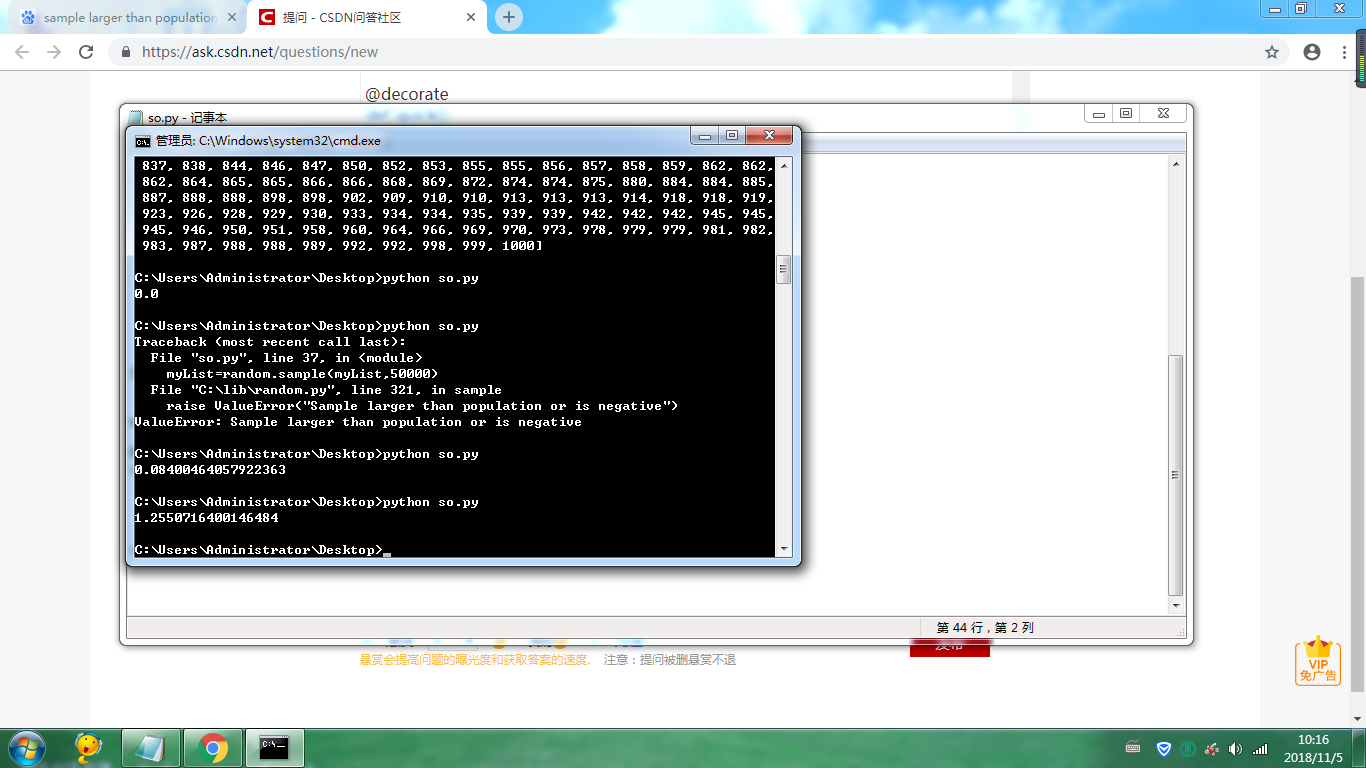python 递归函数的执行时间怎么处理

'''

'''
import time
import random
myList=[]
count=0

def decorate(function):
def inner(*args):
start=time.time()
function(*args)
end=time.time()
print(end-start)
return function
return inner
def QuickSort(myList,start,end):
if start i,j=start,end
base=myList[i]
while i while(i=base):
j=j-1
myList[i]=myList[j]
while(i<j) and (myList[i]<=base):
i=i+1
myList[j]=myList[i]
print(myList)
myList[i]=base
QuickSort(myList,start,i-1)
QuickSort(myList,j+1,end)
return myList

for num in range(0,1000):

``````myList.append(random.randint(0,1000))
``````

myList=random.sample(myList,5)

@decorate
def quick():

``````QuickSort(myList,0,len(myList)-1)
#myList.sort()
``````

quick()

''''''
'''

'''

1个回答PYthon递归函数超过999就开始提示错误

python递归 return 问题

``` class Solution: def canJump(self, nums): if len(nums) == 0: return False def DFS(nums): if len(nums) == 1: return True for i in range(1,nums+1): return DFS(nums[i:]) #上面这两行代码如果改成 return DFS(nums[1:]、 DFS(nums[2:]......)则可以运行，但是引入for循环后，return就变为None) S =Solution() S.canJump([2,0,0]) ``` 想知道怎么解决？卡了我好久

python分别用递归函数和非递归函数的形式实现：输入两个1至10000之间的正整数，输出这两个数之间的所有Fibonacci数列?

names=['john', 'eric', ['cleese','idle'],'micheal'] print_lol(names,0) 对于上面这样一个简单的双重列表，用下面的函数显示它，函数中的参数level值是怎么变化的？按书上的结果就是0 1 0 ，我不理解的是什么时候又从1变到0的？ def print_lol(the_list,level)        for each_item in the_list:        if isinstance(each_item,list):               print_lol(each_item,level+1)        else:               for tab_stop in range(level):                     print("\t",end='')               print(each_item)

python递归的无法返回或者达到某一条件终止并return

``` class Solution: def canJump(self, nums): if len(nums) == 0: return False def DFS(nums): if len(nums) == 1: return True for i in range(1,nums+1): DFS(nums[i:]) if DFS(nums) is False: print('1111') return True elif DFS(nums) is None: print('2222') return False S =Solution() S.canJump([2,0,0]) ``` 两个问题：1为什么我DFS函数没有返回TRUE ，返回的是None 2如果按照网上的方法在 DFS(nums[i:]) 前加上return 为什么我的i不会循环到2这个值，只能是1就停止了？

python：用递归方法编写二分法查找函数 程序报错

python 无法停止递归 求解啊

def dfs1(x,y): show[x][y]='.' for i in f: for j in f: nx=x+i ny=y+j if(nx>=0 and ny>=0 and nx<10 and ny<10 and ans[nx][ny]==0 and(i!=0 or j!=0)and(i==0 or j==0)): dfs1(nx,ny) if(nx>=0 and ny>=0 and nx<10 and ny<10 and ans[nx][ny]!=0 and mine[nx][ny]!=1 and(i!=0 or j!=0)and(i==0 or j==0)): show[nx][ny]=ans[nx][ny] return 0 return 0

Python关于递归的问题

You've recieved a serialized JSON object from an API and have deserialized it using the standard library's json library. The object represents your geneology from a given ancestor downward. Assuming your name is Sally and your given ancestor is Janet, your geneology object would be as follows: geneology_object = { 'husband': 'Craig', 'wife': 'Janet', 'children': { 'Chris': { 'husband': 'Chris', 'wife': 'Jesse', 'children': { 'Rebecca': { 'husband': 'Doug', 'wife': 'Rebecca', } } }, 'Wonda': { 'husband': 'Kevin', 'wife': 'Wonda', 'children': { 'Sally': {} } } } } Write a function with the signature get_generations_down(geneology_object, search_name, generations=0) to recursively search for the number of generations between search_name and the eldest ancestor. If the name is not found, a NameNotFoundError should be raised by the recursive function. Assuming the geneology object above, your function should behave as so: >>> get_generations_down(geneology_object, 'Chris') 1 >>> get_generations_down(geneology_object, 'Sally') 2

python的汉诺塔递归算法

python在递归时使用列表切片【：-1】，回归到上一层时原函数没变，描述的不太清，请看解释，希望有人解惑。

Python习题，递归函数！最后一步不会了！

'''------------------------------------------------------ 【程序填空17】 --------------------------------------------------------- 题目：阅读以下程序并填空，该程序是求阶乘的累加和。 S=0!+1!+2!+.........+n! --------------------------------------------------------- 注意：除要求填空的位置之外，请勿改动程序中的其他内容。 ------------------------------------------------------''' def cal(n): pro=1 #**********SPACE********** for i in range(n): #**********SPACE********** pro=pro*i return pro def main(): n=int(input("请输入一个正整型数值n:")) s=0 #**********SPACE********** for i in range(0,n+1): if i!=n: print("%d! + "%i,end='') else: print("%d! = "%i,end='') #**********SPACE********** s=? print(s) if __name__ == '__main__': main()

python无法打印子目录文件，递归不起作用

import os list_result = os.listdir('.') def search(list_result_copy): for now in list_result_copy: if os.path.isfile(now): # file in current directory print os.path.join(os.path.abspath('.'), now) if os.path.isdir(now): # directory in current directory direction = os.listdir(os.path.join(os.path.abspath('.'), now)) search(direction) #就是这里，我想要递归输出所有子目录文件，但是这里压根儿不执行 if __name__ == '__main__': search(list_result)

public static long pow(long x, int n){ if(n == 0) return 1; if(n == 1) return x; if(n % 2 == 0) return pow(x, n/2) * pow(x, n/2); else return pow(x * x, n/2) * x; } 怎么计算这个方法的运行时间 类似于O(NlagN)的表达形式

npython（numpy）中，怎么正确使用递归？

2019 AI开发者大会

2019 AI开发者大会(AI ProCon 2019)是由中国IT社区CSDN主办的AI技术与产业年度盛会。多年经验淬炼，如今蓄势待发：2019年9月6-7日，大会将有近百位中美顶尖AI专家、知名企业代表以及千余名AI开发者齐聚北京，进行技术解读和产业论证。我们不空谈口号，只谈技术，诚挚邀请AI业内人士一起共铸人工智能新篇章！

MIPS单周期CPU-组成原理实验-华中科技大学

2019数学建模A题高压油管的压力控制 省一论文即代码

2019数学建模A题高压油管的压力控制省一完整论文即详细C++和Matlab代码，希望对同学们有所帮助

Git 实用技巧

C/C++跨平台研发从基础到高阶实战系列套餐

Java基础知识面试题（2020最新版）

Python界面版学生管理系统

Vue.js 2.0之全家桶系列视频课程

linux“开发工具三剑客”速成攻略

JAVA初级工程师面试36问(完结)

java jdk 8 帮助文档 中文 文档 chm 谷歌翻译

JDK1.8 API 中文谷歌翻译版 java帮助文档 JDK API java 帮助文档 谷歌翻译 JDK1.8 API 中文 谷歌翻译版 java帮助文档 Java最新帮助文档 本帮助文档是使用谷

Visual Assist X 破解补丁

vs a's'sixt插件 支持vs2008-vs2019 亲测可以破解，希望可以帮助到大家

150讲轻松搞定Python网络爬虫

【为什么学爬虫？】 &nbsp; &nbsp; &nbsp; &nbsp;1、爬虫入手容易，但是深入较难，如何写出高效率的爬虫，如何写出灵活性高可扩展的爬虫都是一项技术活。另外在爬虫过程中，经常容易遇到被反爬虫，比如字体反爬、IP识别、验证码等，如何层层攻克难点拿到想要的数据，这门课程，你都能学到！ &nbsp; &nbsp; &nbsp; &nbsp;2、如果是作为一个其他行业的开发者，比如app开发，web开发，学习爬虫能让你加强对技术的认知，能够开发出更加安全的软件和网站 【课程设计】 一个完整的爬虫程序，无论大小，总体来说可以分成三个步骤，分别是： 网络请求：模拟浏览器的行为从网上抓取数据。 数据解析：将请求下来的数据进行过滤，提取我们想要的数据。 数据存储：将提取到的数据存储到硬盘或者内存中。比如用mysql数据库或者redis等。 那么本课程也是按照这几个步骤循序渐进的进行讲解，带领学生完整的掌握每个步骤的技术。另外，因为爬虫的多样性，在爬取的过程中可能会发生被反爬、效率低下等。因此我们又增加了两个章节用来提高爬虫程序的灵活性，分别是： 爬虫进阶：包括IP代理，多线程爬虫，图形验证码识别、JS加密解密、动态网页爬虫、字体反爬识别等。 Scrapy和分布式爬虫：Scrapy框架、Scrapy-redis组件、分布式爬虫等。 通过爬虫进阶的知识点我们能应付大量的反爬网站，而Scrapy框架作为一个专业的爬虫框架，使用他可以快速提高我们编写爬虫程序的效率和速度。另外如果一台机器不能满足你的需求，我们可以用分布式爬虫让多台机器帮助你快速爬取数据。 &nbsp; 从基础爬虫到商业化应用爬虫，本套课程满足您的所有需求！ 【课程服务】 专属付费社群+每周三讨论会+1v1答疑

JavaWEB商城项目(包括数据库)

Python数据挖掘简易入门

&nbsp; &nbsp; &nbsp; &nbsp; 本课程为Python数据挖掘方向的入门课程，课程主要以真实数据为基础，详细介绍数据挖掘入门的流程和使用Python实现pandas与numpy在数据挖掘方向的运用，并深入学习如何运用scikit-learn调用常用的数据挖掘算法解决数据挖掘问题，为进一步深入学习数据挖掘打下扎实的基础。

2019 Python开发者日-培训

4小时玩转微信小程序——基础入门与微信支付实战